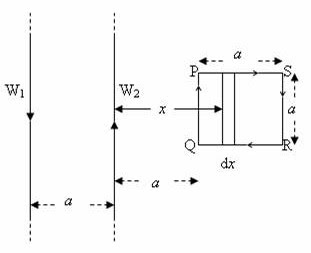## Pages

`“Life is like riding a bicycle.  To keep your balance you must keep moving.”–Albert Einstein`

## Wednesday, October 13, 2010

### AP Physics C – Answer to Free Response Practice Question on Electromagnetic Induction

A free response practice question on electromagnetic induction was posted on 10th October 2010. As promised, I give below a model answer (along with the question) for your benefit.

[You can access earlier posts in this section either by clicking on the label 'electromagnetic induction' below this post or by trying a search for 'electromagnetic induction' using the search box provided on this page].Two infinitely long straight parallel wires W1 and W2, separated by a distance ‘a’ in free space, carry equal currents I flowing in opposite directions as shown in the adjoining figure. A square loop PQRS of side ‘a’, made of nichrome wire of resistance ρ Ω per metre is arranged with its plane lying in the plane of the wires W1 and W2 so that the sides PQ and RS of the loop are parallel to the wires W1 and W2. The side PQ of the loop is at a distance ‘a’ from the wire W2. Now, answer the following questions in terms of the given quantities and fundamental constants:

(a) Determine the magnetic flux density at a point midway between the wires W1 and W2.

(b) Determine the magnetic flux density at the mid point of the square loop PQRS.

(c) Calculate the magnetic flux through the loop PQRS.

(d) What is the average emf induced in the loop when the current through the wires is switched off in a time of 50 ms?

(e) When the current through the wires is switched off, it is found that at a certain instant t, the current decays at the rate of 40 As–1. Calculate the current induced in the loop PQRS at the instant t.

Indicate the direction of the current in the loop and justify your answer.

The magnitude of the magnetic flux density B at a point distant a from an infinitely long straight conductor carrying current I is given by

B = μ0I/2πa where μ0 is the magnetic permeability of free space

The magnetic flux density at a point midway between the wires W1 and W2 is the resultant of the magnetic flux densities produced by these wires. Each wire produces magnetic flux density of magnitude μ0I/2πa.

These fields are directed perpendicular to the plane containing the wires and outwards (towards the reader) and hence they add up to produce a resultant flux density of magnitude μ0I/2πa + μ0I/2πa= μ0I/πa.

[Since the magnetic flux density is a vector, you should not forget to mention its direction].

(b) At the mid point of the square loop PQRS the magnetic fields due to the wires W1 and W2 are directed perpendicular to the plane containing the wires. But the field due to the wire W2 is directed into the plane of the figure (away from the reader) where as the field due to the wire W1 is directed outwards (towards the reader). The resultant field is directed into the plane of the figure (away from the reader) since the wire W2 produces stronger field.

The magnitude of the resultant magnetic flux density at the mid point of the square loop PQRS is [(μ0I)/(2π×3a/2)] – [(μ0I)/(2π×5a/2)]

This is equal to (μ0Ia)[(1/3) (1/5)] = 2μ0I/15πa(c) To find the magnetic flux through the loop PQRS, consider a strip of very small width dx at distance x from the wire W2 as shown in the figure. The resultant magnetic flux density B at the strip is given by

B = 0I/ 2π) [1/x – 1/(a+x)]

[dA is the area of the strip of length a and width dx]

Magnetic flux Ф linked with the entire loop PQRS is given by

Ф = a2a Badx = 0Ia/2π) a2a [1/x – 1/(a+x)]dx

[The limits of integration are x = a and x = 2a].

Therefore, Ф = 0Ia/2π) [ln x – ln (a+x)] between limits x = a and x = 2a.

Or, Ф = = 0Ia/2π) ln [x/(a+x)] between limits x = a and x = 2a.

= 0Ia/2π) [ln(2/3) – ln(1/2)]

= (μ0Ia /2π) ln (4/3) ...............................(i)

[The unit of magnetic flux density is tesla (or, weber per mrtre2) and the unit of magnetic flux is weber].

(d) The average emf Vaverage induced in the loop is given by

Vaverage = Rate of change of magnetic flux

= Change of magnetic flux/ Time

= [(μ0Ia /2π) ln(4/3) – 0]/(50×10–3) since the current through the wires is switched off in a time of 50 ms

Therefore, Vaverage = = (10μ0Ia /π) ln (4/3)

(e) The emf V induced in the loop PQRS at the instant t is given by

V = dФ/dt, the negative sign appearing because of Lenz’s law.

Ignoring the negative sign, we have from equation (i)

V = dФ/dt = [(μ0a /2π) ln (4/3)](dI/dt)

Here dI/dt = 40 As–1, as given in the question.

Substituting for dI/dt, we have V = (20μ0a /π) ln (4/3)

The induced current in the loop = V/R where R is the resistance of the loop, which is equal to 4aρ ohm.

Therefore, induced current = (20μ0a /4π) ln (4/3) = (5μ0 ρ) ln (4/3) ampere.

The direction of the induced current in the loop is clockwise, as indicated in the figure.

The resultant magnetic field is directed normally into the plane of the loop and is decreasing when the current is switched off. The induced current should oppose this change and should therefore produce a magnetic field acting in the same direction (normally into the plane of the loop). This is made possible by the clockwise flow of the induced current.